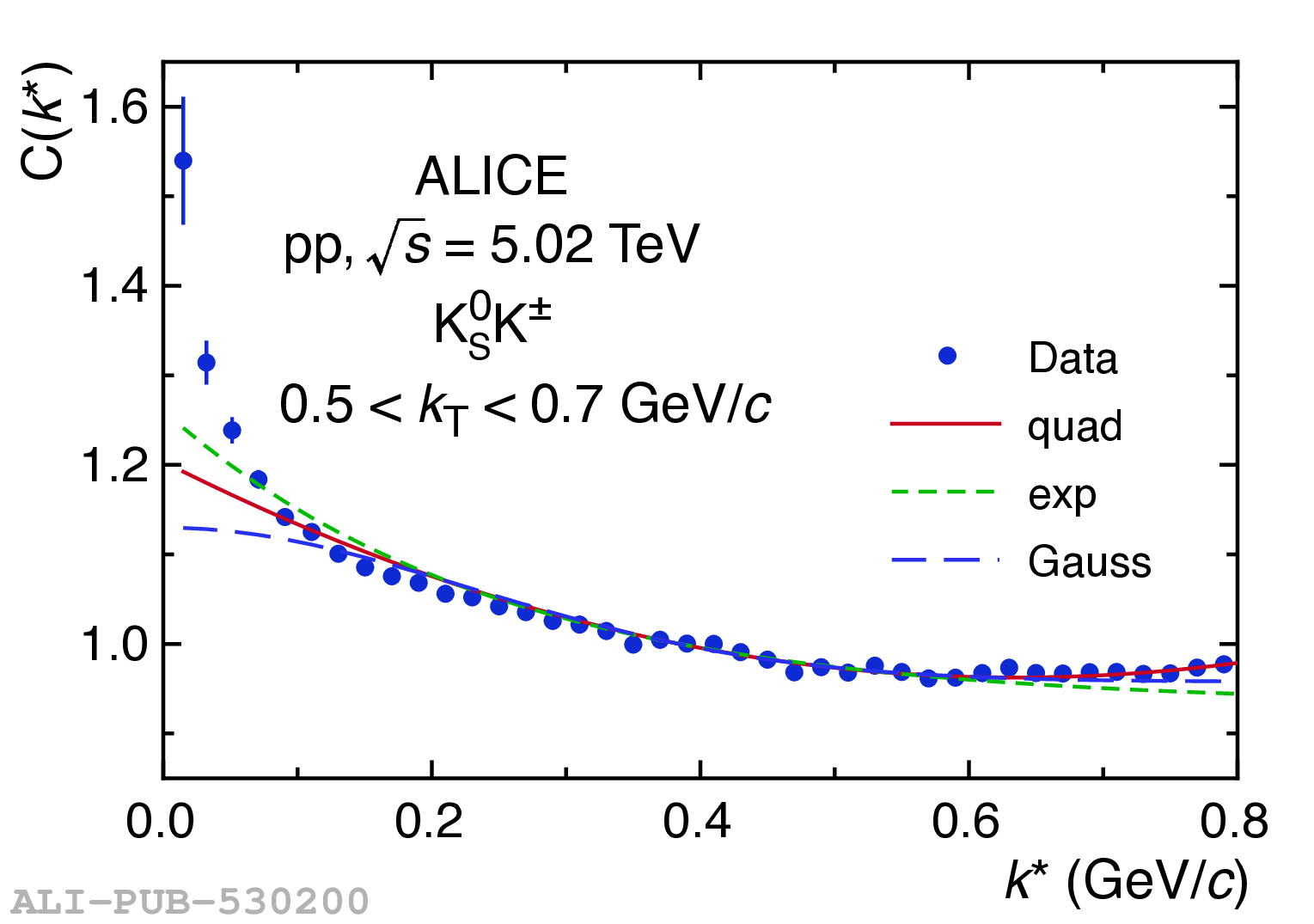# Figure 2

 Example K$^0_{\rm S}$K$^\pm$ correlation functions plotted with fits of Eqs. 3, 4 and 5 in pp collisions at $\sqrt{s}=5.02$ (left) and $13$ TeV (right).